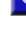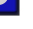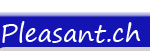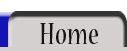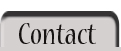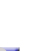All OperatorsOPERATOR:  Not Implemented in version 1.0  Not   The Not operator is used to perform a logical negation on an expression. The expression must be of Boolean subtype and have a value of True or False. This operator causes a True expression to become False, and a False expression to become True.   The Not operator can also be used a "bitwise operator" to make a bit-by-bit inversion of the binary values of an integer. If the bit is a 1, then a 0 is returned. If the bit is a 0, then a 1 is returned. Thus, the binary value 101 becomes 010.   Code: <% AnyExpression = True %> <% =Not AnyExpression %>   Output: False